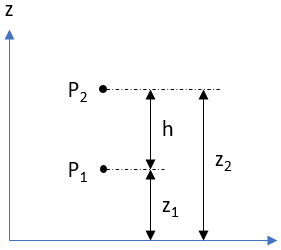## Pressure Difference

At 10,994 meters below sea level, the Mariana Trench is the deepest known point in the Earth’s ocean. If a submarine is exploring the ocean depths 2,500 m above the Trench’s floor, what is the difference in pressure between the submarine and sea level? Assume the density of salt water is 1,026 kg/m^3 and is constant throughout the ocean’s depths.

Hint
The pressure difference between two different points:
$$P_2-P_1=-\gamma(z_2-z_1)=-\gamma h=-\rho gh$$$where $$\gamma$$ is the liquid’s specific weight, $$\rho$$ is the liquid’s density, and $$g$$ is the acceleration due to gravity Hint 2 $$P_2$$ , $$P_1$$ , $$z_1$$ , $$z_2$$ , and $$h$$ are defined as:The pressure difference between two different points: $$P_2-P_1=-\gamma(z_2-z_1)=-\gamma h=-\rho gh$$$
where $$\gamma$$ is the liquid’s specific weight, $$\rho$$ is the liquid’s density, $$g$$ is the acceleration due to gravity, and $$P_2$$ , $$P_1$$ , $$z_1$$ , $$z_2$$ , and $$h$$ are defined as:Based on the problem statement, the submarine is at $$P_1$$ and sea level is at $$P_2$$ . It also gives $$z_1$$ and $$z_2$$ for the fact about the Mariana Trench’s depth and the submarine’s location. Thus,
$$P_2-P_1=\rho gh=\rho g(z_2-z_1)=(1,026\frac{kg}{m^3})(9.8\frac{m}{s^2})(10,994m-2,500m)$$$$$\Delta P=10,054.8\frac{N}{m^3}(8,494m)=85,405,471\frac{N}{m^2}=85\:MPa$$$
85 MPa